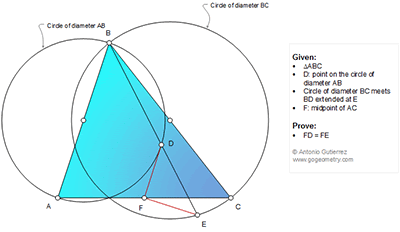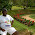## Saturday, November 14, 2015

### Geometry Problem 1166: Triangle, Circles, Diameters, Secant, Midpoint, Congruence

Geometry Problem. Post your solution in the comments box below.
Level: Mathematics Education, High School, Honors Geometry, College.

Click the figure below to view more details of problem 1166.1.Take X - reflection of E in F, AECX is a parallelogram, so AX||CE, i.e. AX_|_BE, A-X-D collinear, XED is right-angled triangle, thus EF=FX=DF, done.

Best regards

1.Excellent work Stan!

With your permission a slightly modified approach...,,,,,

Let EF meet AD at X then Tr.s AXF & CEX are congruent ASA

So XF = FE and so F is the centre of right Tr. XDE and hence DF = FE

2.Corollary: Let BF(extended) meet the circles at X,Y.Then XY is bisected at F.

3.Call other intersection of the 2 circles B' which is the foot of altitude from B.
Let EF and AD meet at P. Then <PAF=<DBB'=<ECB', which means that AP||EC.
From congruent triangles APF and CEF, PF=FE, which makes F circumcenter of right triangle PDE.